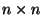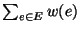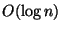Next: MINIMUM PARTITION OF RECTANGLE Up: Miscellaneous Previous: MINIMUM K-LINK PATH IN   Index

### MINIMUM SIZE ULTRAMETRIC TREE

• INSTANCE:matrix M of positive integers.

• SOLUTION: An ultrametric tree, i.e., an edge-weighted tree T(V,E) with n leaves such that, for any pair of leaves i and j,wheredenotes the sum of the weights in the path between i and j.

• MEASURE: The size of the tree, i.e.,where w(e) denotes the weight of edge e.

• Bad News: Not approximable withinfor some.

• Comment: Transformation from graph coloring. Variation in which M is a metric is approximable within.

Viggo Kann
2000-03-20